Next Continued Fraction Arithmetic 29

# Output

• Now suppose that someone asks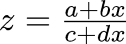to output a term

• Say it decides its next term is q, which it emits

• (We'll soon see how it decides this)

• Then it turns into z', where z = q + 1/z', so z' = 1/(z - q)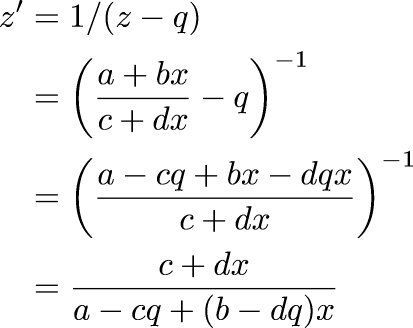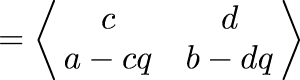• So if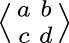is asked for an output and emits q, it turns into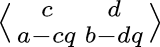• "Egestion"

 Next Back